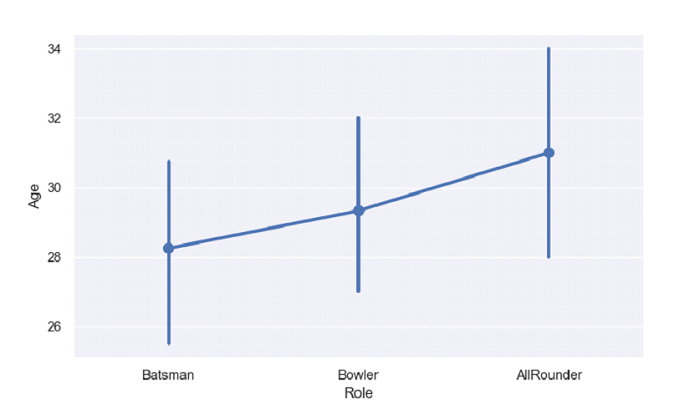# Python Pandas - Draw a set of vertical point plots grouped by a categorical variable with Seaborn

Point Plot in Seaborn is used to show point estimates and confidence intervals using scatter plot glyphs. The seaborn.pointplot() is used for this. For vertical point plot grouped by a categorical variable, set the variable as a value for the pointplot().

Let’s say the following is our dataset in the form of a CSV file − Cricketers.csv

At first, import the required libraries −

import seaborn as sb
import pandas as pd
import matplotlib.pyplot as plt

Load data from a CSV file into a Pandas DataFrame −

dataFrame = pd.read_csv("C:\Users\amit_\Desktop\Cricketers.csv")


Vertical point plot grouped by a categorical variable −

sb.pointplot(dataFrame['Role'],dataFrame['Age'])

## Example

Following is the code −

import seaborn as sb
import pandas as pd
import matplotlib.pyplot as plt

# Load data from a CSV file into a Pandas DataFrame

sb.set_theme(style="darkgrid")

# vertical point plot grouped by a categorical variable
sb.pointplot(dataFrame['Role'],dataFrame['Age'])

# display
plt.show()

## Output

This will produce the following output −Updated on: 30-Sep-2021

109 Views